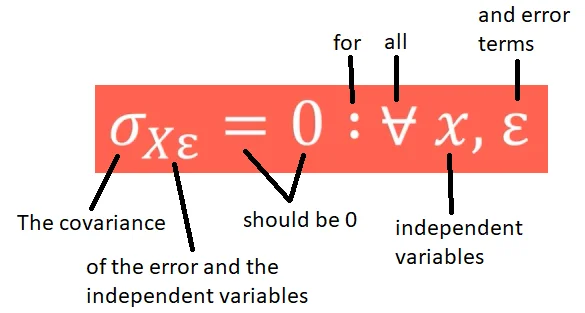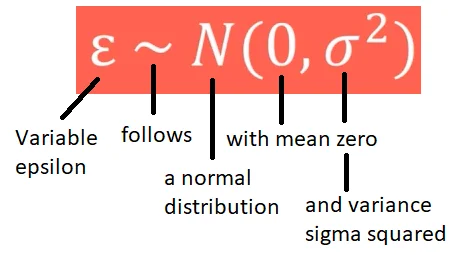05 Jan 2023

Posted on:

28 Dec 2022

0

# Resolved:No endogeneity equition

I have a hard time understanding the equation  or the letters/signs used in it. Could you help to explain?

Thanks

Lu

Posted on:

03 Jan 2023

0

Hey Lu,

Thank you for reaching out!

In mathematics, it's very common to use Greek letters as symbols representing variables. Let me address each of the assumptions independently.

1. In the Linearity assumption, you can see the lowercase Greek letters gamma (the dependent variable), beta (the coefficients), and epsilon (the error term). More on the meaning of each of these symbols you can find on page 3 of the course notes. The linear regression model itself is discussed in "The linear regression model" lecture.

2. In the No endogeneity assumption, you can find the lowercase Greek letters sigma (denoting covariance) and epsilon (denoting the error term). The colon in the expression should be read as "for" and the symbol that resembles an upside down A should be read as "all". Therefore, the expression should be read as follows:
"The covariance of the error and the independent variables should be 0 for all independent variables and error terms."3. The notation used in the expression of the third assumption makes use of the lowercase Greek letters epsilon and sigma. You can also see the symbol tilde which should be read as "follows". The entire expression should therefore be read as:
"Variable epsilon follows a normal distribution with mean zero and variance sigma squared."For an in-depth discussion on the topic, please refer to the "Continuous Distributions" section of our Probability course.

4. The expression in the fourth assumption should be read as follows:
"The covariance (sigma) between any two error terms (epsilon i and epsilon j) should be zero for all values of i and j, where i and j are not equal to each other."

5. In the expression representing the final fifth assumption, you can see the lowercase Greek letter rho (representing correlation), as well as a symbol resembling a wavy equal sign. The latter should be read as "approximately equal to". When a line is crossing the symbol, as in the expression, it instead reads "not approximately equal to". Therefore, the entire expression reads as follows:
"The correlation between two independent variables (x i and x j) should not be approximately equal to 1 for all values of i and j, where i is different from j."

We understand that mathematical notation can be tedious to read at first. However, once you get the hang of it, you will find out that it's a great way of expressing statements in a compact way.

Hope this helps!

Kind regards,
365 Hristina

Posted on:

05 Jan 2023

0

Thanks a lot for the detailed explanation. This helps a lot!

Lu